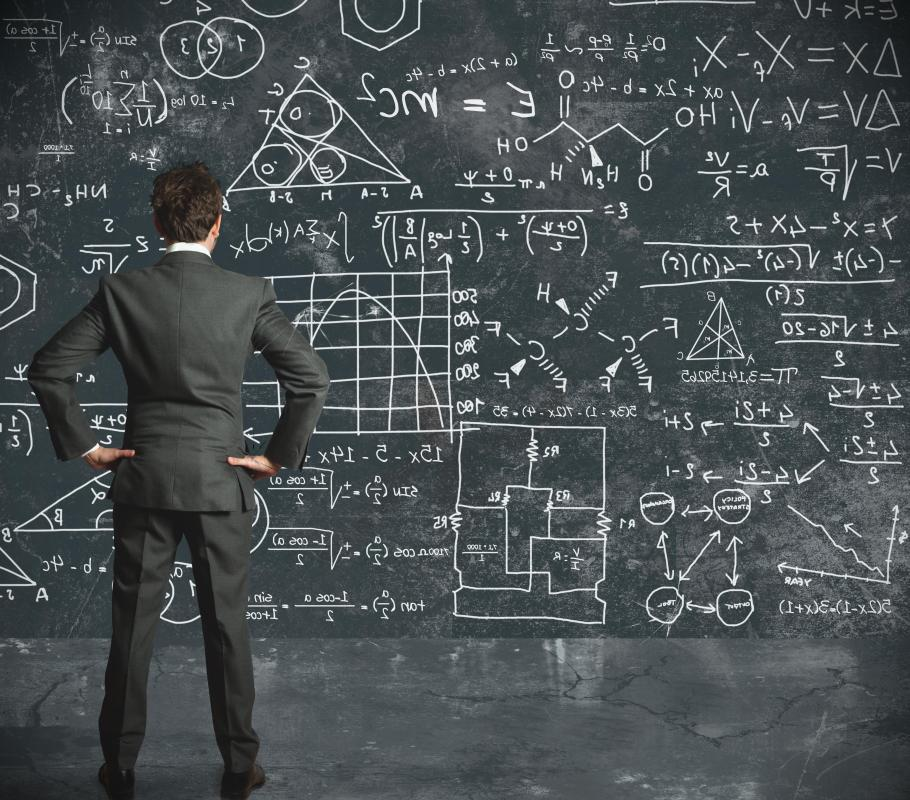# What is an Applied Force?

Alex Paul
Alex PaulThe scientific term "force" refers to an interaction between two or more objects.

The scientific term "force" refers to an interaction between two or more objects. Theoretically, the phrase "applied force" could be used for any force, but it usually is used when talking about a contact force between a person and an object. In the case of a book being picked up, for example, the applied force would be the force providing the upward acceleration by the person.

There are two broad categories of forces: those that act at a distance and those that act because of contact between two objects. As the nature of an applied force specifies that it is caused by a person, in most cases it is a contact force. There are exceptions to this, though, such as the gravitational interaction between a human and an object, although this is almost always negligible.

Applied forces are often difficult to calculate because they are usually found in conjunction with other forces. The only exception to this would be if the force is taking place in a vacuum with no bodies exerting a gravitational force. Applied force physics usually focuses on finding the total resultant force acting on an object rather than separating the components of the force. This is useful because the subsequent movement of an object only depends on the direction and strength of the resultant force.

When calculating the future movement of an object, the applied forces are neither the only consideration nor the most important. All forces are measured in Newtons and the overall movement does not depend on the source of the force, only the direction and strength. For this reason, there is no universal applied forces formula. When calculating an applied force, the applied force equation will depend on the situation. If other variables, such as mass and acceleration, are given, however, then the applied force may be calculated using the standard equation F=MxA — M refers to the mass of the accelerating object and A is the acceleration.

In many cases, there will be an opposing force that acts against the force applied by a person. Friction, for example, is commonly found to act in the opposite direction. If a calculation of resultant force is being performed using an applied force, friction must be included in the calculation. In other situations, gravity or air resistance might oppose applied forces. This is why whenever a force is performed by a human that an amount of work that is equal to the energy gained is done on the object.

## You might also Likesherlock87

Whenever I hear about gravity, I think of a quote from Big Bang Theory. In it, the physicist Leonard says to his fellow physicist and roommate Sheldon, "You are not Isaac Newton." And Sheldon replies, "No, no, that's true. Gravity would have been apparent to me without the apple."

I have always wondered about the story about the apple, and I hope it really was obvious to Newton with the apple as well.

widget2010

@rosoph, better yet, without gravity we might not exist in a world with an atmosphere at all, in which case we wouldn't even be here to discuss the application of force.

Science is weird.

rosoph

Imagine what it would be like to use applied force, without gravity being a force of opposition! A human being would be able to use applied force to pick up anything -- even a car!

On the other hand, if gravity did not exist, we wouldn't really have to use applied force to actually pick up anything, because everything would just be floating around, like in space. In this case, the applied force we used would simply be to push things around, or maybe pull them to us.